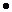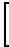edHelper subscribers - Create a new printable

Sample edHelper.com - Exponents Worksheet

 Name _____________________________Date ___________________
Exponents
1.
 5(71) =

2.
 5h(7hh) =

 3

4.
 (28)= 144

5.
 (2j8j)= 144j3

6.
 eeeee =

7.
 8(56) =

 8

9.+ c + c + c + c = 5c

10.
 9j + 9j + 9j + 9j += 45j

 11

12.
 5(4 + 8) =

13.
 5e(4e + 8e) =

 14

Evaluate to a single number.
 15 23
 16 62
 17 50

Evaluate to a single number.
18.
 50 + 83

19.
 23 + 74

Simplify.
20.
 4-8 × 4-5

21.
 34 × 36

Simplify.
 22 (16x)3  (32x)2
 23 (-5x)0

Simplify.
24.98(-9)-6÷  9-6

25.512(-5)3Simplify.
 26 (-4x)    ÷  (-9x)3
 27 (32x)2    ÷  (22x)0

Simplify.
 28 (-9h3q6)(-5h5)
 29 (-l4)(12l3n5)

Z represents a missing exponent. What value should Z be?
30.
 (-2q4)(-8qZo6) = 16q10o6

31.
 (-8l4a6)(10l2)(3lZ) = -240l9a6

Simplify.
 32 (-8g-6o6)(-10g3)(3g4o-4)
 33 (-8d-3v-3)(-4d-5v-5)(d-3)

Z represents a missing exponent. What value should Z be?
34.
 (6y3t3)(-8yZt-2) = -48y5t

35.
 (-9o-2w2)(-9o3w3)(5oZ) = 405o-3w5

Simplify.
 36 (-5s-5q5)5(-s-6q6)5
 37 (2y3)4

Z represents a missing exponent. What value should Z be?
38.
 (-z-2w2)4(z-2)Z = z-16w8

39.
 (2p-4)Z = 16p-16

Simplify.
40.
 -5v5b2-8v6b3

41.
 -5d2o37d3o6

Z represents a missing exponent. What value should Z be?
42.
 -4z6-12zZf6

=
 z3f6

43.
 6pZs37p6s2

=
 6s7

Simplify.
44.
 (-10r6o2)(-5r5o3)2r2

45.
 -d5(-11d3)(-2d2t3)

Z represents a missing exponent. What value should Z be?
46.
 -7w3x59w6-9w2-8wZ

=
 -7x58w5

47.
 -10t5v6(11t3vZ)(11t3v6)

=
 -10121tv3

Simplify.
48.
 (-q3o5)(-3)2-4q5o3

49.
 (-12m5i5)(2)55m6

Z represents a missing exponent. What value should Z be?
50.
 9jZ(-3j2x2)5

=
 -127j7x10

51.
 -11a4(-9a2)(2aZe6)3

=
 1172a7e18

Rewrite the number in scientific notation.
 52 3,000,000
 53 9.52e-12

Rewrite the number in decimal notation.
 54 4.64 x 1012
 55 8.2 x 10-12

Simplify and write the answer in scientific notation.
 56 (4.1 x 10-7)  -  (3.4 x 10-8)
 57 (3.6 x 107)(6 x 10-5)

Simplify and write the answer in scientific notation.
58.
 5 x 1054 x 10-5

59.
 (7 x 105)(8 x 10-6)4 x 107

Sample
This is only a sample worksheet.

edHelper subscribers - Create a new printable